## Homework Problems

1. Show that the Hamiltonian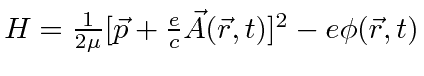yields the Lorentz force law for an electron. Note that the fields must be evaluated at the position of the electron. This means that the total time derivative ofmust also account for the motion of the electron.

2. Calculate the wavelengths of the three Zeeman lines in the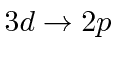transition in Hydrogen atoms in agauss field.

3. Show that the probability flux for system described by the Hamiltonian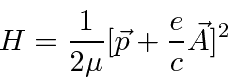is given byRemember the flux satisfies the equations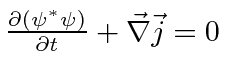.

4. Consider the problem of a charged particle in an external magnetic fieldwith the gauge chosen so that. What are the constants of the motion? Go as far as you can in solving the equations of motion and obtain the energy spectrum. Can you explain why the same problem in the gaugesand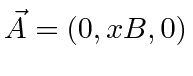can represent the same physical situation? Why do the solutions look so different?

5. Calculate the top left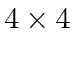corner of the matrix representation offor the harmonic oscillator. Use the energy eigenstates as the basis states.

6. The Hamiltonian for an electron in a electromagnetic field can be written as. Show that this can be written as the Pauli HamiltonianJim Branson 2013-04-22